# DI Table Graph and Chart Questions For Bank PO Exams

Question No. 1

Directions: Answer the questions based on the information given below.

The given table shows the total number of boilers (Taap and Bhaap), average quantity of water boiled per boiler, percentage of the quantity of water boiled in Taap out of total quantity of water boiled in both the boilers together, average quantity of water boiled per Bhaap, in four different factories.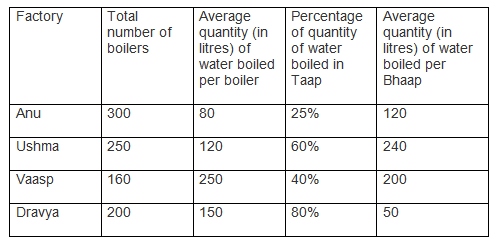For Anu, find the ratio of the average quantity of water boiled in each Taap and in each Bhaap.

A – 3:4

B – 2:5

C – 1:3

D – 5:7

E- None of these

Solution

For Anu:

Sum of quantities of water boiled in given two type of boilers = 80 x 300 = 24000 litres

Quantity of water boiled in Taap = 0.25 x 24000 = 6000 litres

Quantity of water boiled in Bhaap = 24000 – 6000 = 18000 litres

Total number of Bhaap = 18000/120 = 150

Total number of Taap = 300 – 150 = 150

Average quantity of water boiled per Taap = 6000/150=40 litres

Similarly,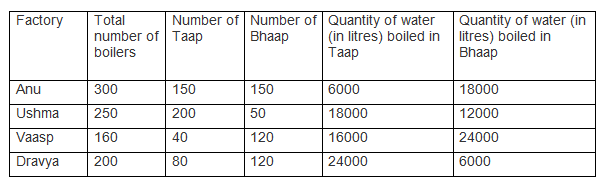Average of quantity of water boiled in each Taap = 6000/150 = 40 litres

Average of quantity of water boiled in each Bhaap = 120 litres

Required ratio = 40:120 = 1:3

Hence, option C.

Question No. 2

Directions: Answer the questions based on the information given below.

The given table shows the total number of boilers (Taap and Bhaap), average quantity of water boiled per boiler, percentage of quantity of water boiled in Taap out of total quantity of water boiled in both the boilers together, average quantity of water boiled per Bhaap, in four different factories.Number of Bhaap in Ushma is how much percent more/less then number of Taap in it?

A- 75%

B- 60%

C- 80%

D- 45%

E- None of these

Solution

For Anu:

Sum of quantities of water boiled in given two type of boilers = 80 x 300 = 24000 litres

Quantity of water boiled in Taap = 0.25 x 24000 = 6000 litres

Quantity of water boiled in Bhaap = 24000 – 6000 = 18000 litres

Total number of Bhaap = 18000/120 = 150

Total number of Taap = 300 – 150 = 150

Average quantity of water boiled per Taap = 6000/150=40 litres

Similarly,Required percentage = {(200 – 50)/200} x 100 = 75%

Hence, option A

Question No. 3

Directions: Answer the questions based on the information given below.

The given table shows the total number of boilers (Taap and Bhaap), average quantity of water boiled per boiler, percentage of quantity of water boiled in Taap out of total quantity of water boiled in both the boilers together, average quantity of water boiled per Bhaap, in four different factories.On a certain day in Vaasp, 25% of number of Taap and 1/3rd of the number of Bhaap were not working. If the average quantity of water boiled in each Taap and each Bhaap on that day was 60 litres and 50 litres, respectively then find the total quantity of water boiled in boilers in Vaasp.

A – 6200 litres

B – 4800 litres

C- 6000 litres

D – 5400 litres

E – None of these

Solution

For Anu:

Sum of quantities of water boiled in given two type of boilers = 80 x 300 = 24000 litres

Quantity of water boiled in Taap = 0.25 x 24000 = 6000 litres

Quantity of water boiled in Bhaap = 24000 – 6000 = 18000 litres

Total number of Bhaap = 18000/120 = 150

Total number of Taap = 300 – 150 = 150

Average quantity of water boiled per Taap = 6000/150=40 litres

Similarly,Number of Taap working in Vaasp = 0.75 x 40 = 30

Quantity of water boiled in Taap = 30 x 60 = 1800 litres

Number of Bhaap working in Vaasp = (2/3) x 120 = 80

Quantity of water boiled in Bhaap = 80 x 50 = 4000 litres

Required quantity of water boiled = 4000 + 1800 = 5800 litres

Hence, option E.

Question No. 4

Directions: Answer the questions based on the information given below.

The given table shows the total number of boilers (Taap and Bhaap), average quantity of water boiled per boiler, percentage of quantity of water boiled in Taap out of the total quantity of water boiled in both the boilers together, average quantity of water boiled per Bhaap, in four different factories.In Dravya, 20 more number of Taap and 25% more number of Bhaap, were installed. Find the difference between new number of Taap and Bhaap in Dravya.

A – 20

B – 50

C-40

D-60

E – None of these

Solution

For Anu:

Sum of quantities of water boiled in given two type of boilers = 80 x 300 = 24000 litres

Quantity of water boiled in Taap = 0.25 x 24000 = 6000 litres

Quantity of water boiled in Bhaap = 24000 – 6000 = 18000 litres

Total number of Bhaap = 18000/120 = 150

Total number of Taap = 300 – 150 = 150

Average quantity of water boiled per Taap = 6000/150=40 litres

Similarly,New number of Taap = 80 + 20 = 100

New number of Bhaap = 1.25 x 120 = 150

Required difference = 150 – 100 = 50

Hence, option B

Question No. 5

Directions: Answer the questions based on the information given below.

The given table shows the total number of boilers (Taap and Bhaap), average quantity of water boiled per boiler, percentage of quantity of water boiled in Taap out of total quantity of water boiled in both the boilers together, average quantity of water boiled per Bhaap, in four different factories.Find the sum of average quantity of water boiled per Taap in Ushma and Dravya.

A – 420 litres

B – 360 litres

C- 280 litres

D – 390 litres

E. None of these

Solution

For Anu:

Sum of quantities of water boiled in given two type of boilers = 80 x 300 = 24000 litres

Quantity of water boiled in Taap = 0.25 x 24000 = 6000 litres

Quantity of water boiled in Bhaap = 24000 – 6000 = 18000 litres

Total number of Bhaap = 18000/120 = 150

Total number of Taap = 300 – 150 = 150

Average quantity of water boiled per Taap = 6000/150=40 litres

Similarly,Required sum = 90 + 300 = 390 litres

Hence, option D.

Question No. 6

Directions: Answer the questions based on the information given below.

The given table shows the data regarding the number of air conditioners and air coolers sold by a shop in 5 different months and also percentage of those installed.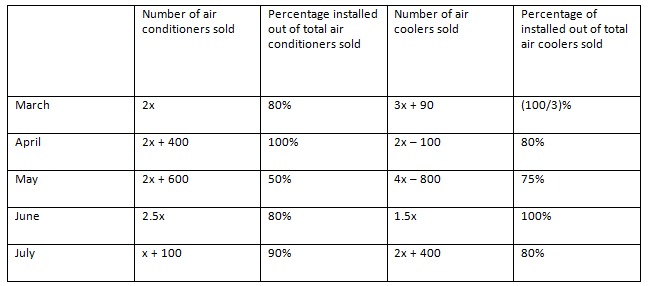If total number of air conditioners installed in all the months together is 1540 units, then find out the number of air coolers installed in the month of June.

A – 120

B – 170

C – 140

D – 210

E – 150

Solution

In March, Number of air conditioners installed = 2x * 0.8 = 1.6x

Number of air coolers installed = (3x + 90) * 1/3 = x + 30

Similarly, we can find out the number of air conditioners and air coolers installed in the given 5 months.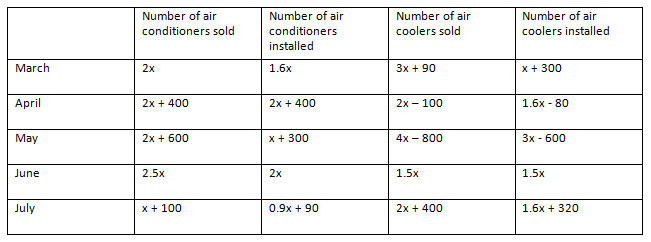Total number of air conditioners installed in all the months together

= (1.6x) + (2x + 400) + (x + 300) + 2x + (0.9x + 90)

= 7.5x + 790

According to the question,

7.5x + 790 = 1540

Or, 7.5x = 750

Or, x = 100

Number of air coolers installed in the month of June = 1.5 x 100 = 150

Hence, option E.

Question No. 7

Directions: Answer the questions based on the information given below.

The given table shows the data regarding the number of air conditioners and air coolers sold by a shop in 5 different months and also percentage of those installed.If number of air conditioners installed in April and May together is 150 more than the number of air coolers sold in June and July together, then find out the total number of air conditioners sold in March, April and July together.

A – 1200

B – 1700

C – 2000

D – 2100

E – 1500

Solution

In March, Number of air conditioners installed = 2x * 0.8 = 1.6x

Number of air coolers installed = (3x + 90) * 1/3 = x + 30

Similarly, we can find out the number of air conditioners and air coolers installed in the given 5 months.Number of air conditioners installed in April and May together = (2x + 400) + (x + 300) = 3x + 700

Number of air coolers sold in June and July together = (1.5x) + (2x + 400) = 3.5x + 400

According to the question,

(3x + 700) – (3.5x + 400) = 150

Or, -0.5x + 300 = 150

Or, 0.5x = 150

Or, x = 300

Total air conditioners sold in March, April and July together

= (2x) + (2x + 400) + (x + 100)

= 5x + 500

= 2000

Hence, option C.

Question No. 8

Directions: Answer the questions based on the information given below.

The given table shows the data regarding the number of air conditioners and air coolers sold by a shop in 5 different months and also percentage of those installedFind the difference between number of air conditioners installed in April and June together and the number of air coolers installed in March and May together.

A – Can’t be determined

B – 970

C- 870

D – 210

E – 530

Solution

In March, Number of air conditioners installed = 2x * 0.8 = 1.6x

Number of air coolers installed = (3x + 90) * 1/3 = x + 30

Similarly, we can find out the number of air conditioners and air coolers installed in the given 5 months.Number of air conditioners installed in April and June together = (2x + 400) + 2x = 4x + 400

Number of air coolers installed in March and May together = (x + 30) + (3x – 600) = 4x – 570

Required difference = (4x + 400) – (4x – 570) = 970

Hence, option B.

Question No. 9

Directions: Answer the questions based on the information given below.

The given table shows the data regarding the number of air conditioners and air coolers sold by a shop in 5 different months and also percentage of those installed.If the ratio between number of air conditioners installed in July to the air coolers installed in March is 3:2, then find out the total number of air conditioners and air coolers together sold in the month of July.

A – 725

B – 975

C- 775

D- 750

E – 580

Solution

In March, Number of air conditioners installed = 2x * 0.8 = 1.6x

Number of air coolers installed = (3x + 90) * 1/3 = x + 30

Similarly, we can find out the number of air conditioners and air coolers installed in the given 5 months.According to the question,

(0.9x + 90):(x + 30) = 3:2

Or, 3x + 90 = 1.8x + 180

Or, 1.2x = 90

Or, x = 75

The total number of units sold in the month of July

= (x + 100) + (2x + 400)

= 3x + 500

= 3 × 75 + 500

= 725

Hence, option A.

Question No. 10

Directions: Answer the questions based on the information given below.

The given table shows the data regarding the number of air conditioners and air coolers sold by a shop in 5 different months and also percentage of those installed.If average number of air conditioners sold in the months of April and May is 600, then what is the average of the number of air coolers sold in the months of June and July?

A – 289.5

B – 235.5

C – 287.5

D- 275.5

E – 290.5

Solution

In March, Number of air conditioners installed = 2x * 0.8 = 1.6x

Number of air coolers installed = (3x + 90) * 1/3 = x + 30

Similarly, we can find out the number of air conditioners and air coolers installed in the given 5 monthsAverage number of air conditioners sold in the months of April and May = {(2x + 400) + (2x + 600)}/2 = 2x + 500

According to the question,

2x + 500 = 600
Or, 2x = 100

Or, x = 50

Now, average of the number of air coolers sold in the months of June and July

= {(1.5x) + (2x + 400)}/2

= (3.5x + 400)/2

= (3.5 x 50 + 400)/2

= 575/2

= 287.5

Hence, option C

Question No. 11

Direction: Answer the questions based on the information given below:

The given table shows the number of cooler sold ad ratio of number of coolers sold to the number of ACs sold, in five different cities.

Note: Total product sold = Number of (coolers + ACs) sold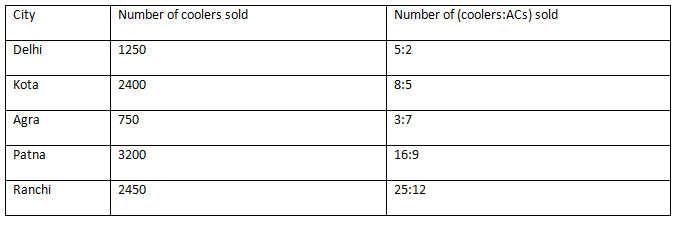Find the ratio of total product sold in Delhi to number of ACs sold in Agra

A – 4:5

B – 3:2

C – 1:1

D – 2:1

E – 5:3

Solution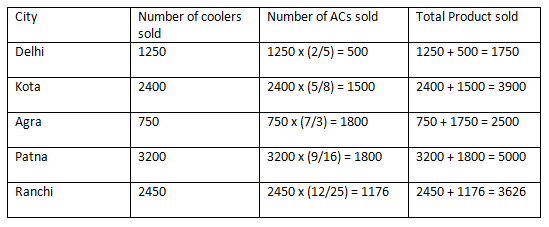Required ratio = 1750:1750 = 1:1

Hence, option C

Question No. 12

Direction: Answer the questions based on the information given below:

The given table shows the number of cooler sold ad ratio of number of coolers sold to the number of ACs sold, in five different cities.

Note: Total product sold = Number of (coolers + ACs) soldTotal number of product sold in Ranchi is how much percent more/less than that in Patna?

A – 27.48%

B – 24.56%

C – 29.12%

D – 23.48%

E – None of these

SolutionRequired percentage = {(5000 – 3626)/5000} x 100 = 27.48%

Hence, option A

Question No. 13

Direction: Answer the questions based on the information given below:

The given table shows the number of cooler sold ad ratio of number of coolers sold to the number of ACs sold, in five different cities.

Note: Total product sold = Number of (coolers + ACs) soldFind the average number of ACs sold in Delhi and Agra

A – 1125

B – 1245

C – 1315

D – 1095

E – 975

SolutionRequired average = (500 + 1750)/2 = 1125

Hence, option A

Question No. 14

Direction: Answer the questions based on the information given below:

The given table shows the number of cooler sold ad ratio of number of coolers sold to the number of ACs sold, in five different cities.

Note: Total product sold = Number of (coolers + ACs) soldOut of total products in Agra, 40% were of LG and rest of Haier. If 2/3rd of coolers sold in Agra were of Haier, then find the number of ACs of Haier sold in Agra.

A – 750

B – 1050

C – 540

D – 950

E – 1000

SolutionTotal products of Haier sold in Agra = 0.6 x 2500 = 1500

Number of coolers of Haier sold in Agra = (2/3) x 750 = 500

Therefore, number of ACs of Haier sold in Agra = 1500 – 500 = 1000

Hence, option E

Question No. 15

Direction: Answer the questions based on the information given below:

The given table shows the number of cooler sold ad ratio of number of coolers sold to the number of ACs sold, in five different cities.

Note: Total product sold = Number of (coolers + ACs) soldIf the ratio of the number of ACs sold in Patna and Ambala is 9:5, respectively, then find the number of coolers sold in Ambala which is 200 less than that the number of ACs sold in the same city.

A – 750

B – 800

C – 1200

D – 600

E – None of theses

SolutionNumber of ACs sold in Ambala = 1800 x (5/9) = 1000

Number of coolers sold in Ambala = 1000 – 200 = 800

Hence, option B

Question No. 16
Directions: Answer the questions based on the information given below.

Different number of articles (bracelets, boxes and pens) was sold in five different months. The given table shows the total number of articles sold, number of bracelets sold and ratio of number of boxes to pens sold, in given five months.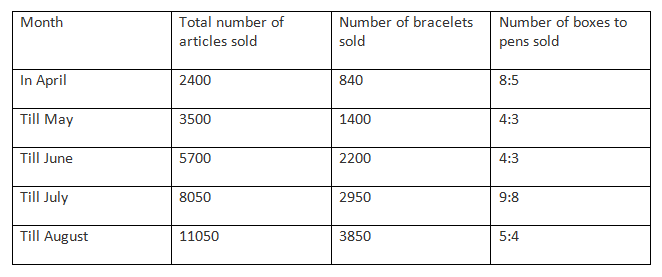Out of total number of pens sold in May and June together 40% were red pens and rest were black pens. If the cost of each red pens and each black pens sold in given two months are Rs. 5 and Rs. 3 respectively then find the difference between amount earned by selling red and black pens in given two months together.

A –  Rs. 180

B –  Rs. 240

C – Rs. 200

D –  Rs. 160

E –  None of these

Solution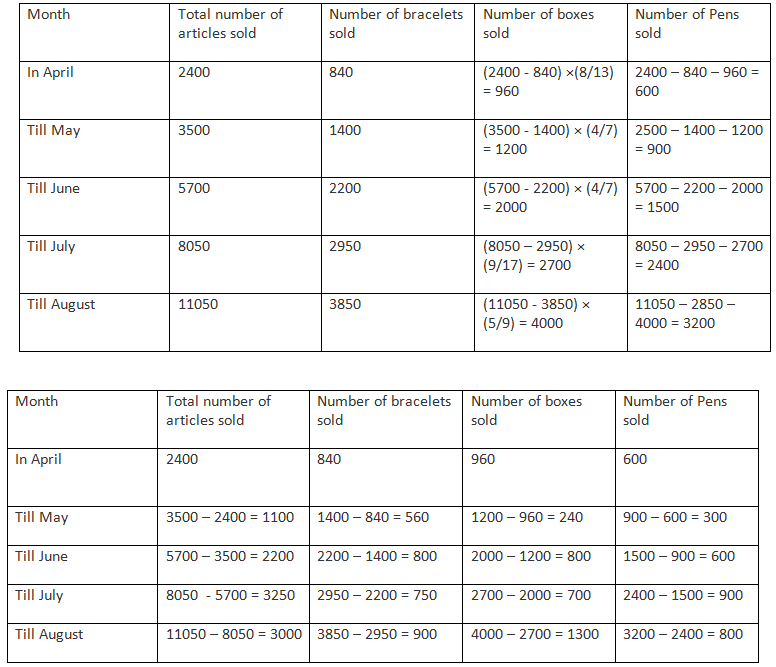Number of black pens sold in May and June together = 0.6 × (600 + 300) = 540

Number of red pens sold in May and June together = 0.4 × (600 + 300) = 360

Required difference = 360 × 5 – 540 × 3 = 1800 – 1620 = Rs. 180

Hence, option A

Question No. 17
Directions: Answer the questions based on the information given below.

Different number of articles (bracelets, boxes and pens) was sold in five different months. The given table shows the total number of articles sold, number of bracelets sold and ratio of number of boxes to pens sold, in given five months.In July, 1/15th of number of bracelets, 50% of number of boxes and 60% of number of pens were sold to males and rest to females. How much percent of articles were sold to females in July?

A –  50%

B –  20%

C –  40%

D –  60%

E –  None of these

SolutionIn July,

Number of articles sold to males = (750/15) + (700/2) + (0.6 × 900) = 50 + 350 + 540 = 940

Required percentage = {(2350 – 940)/2350} × 100 = 60%
Hence, option D

Question No. 18
Directions: Answer the questions based on the information given below.

Different number of articles (bracelets, boxes and pens) was sold in five different months. The given table shows the total number of articles sold, number of bracelets sold and ratio of number of boxes to pens sold, in given five months.In April, May and July each bracelet was sold for Rs. 8, Rs. 10 and Rs. 5 respectively. If the total amount earned by selling bracelets in given three months is equal to the amount earned by selling boxes in July and August together then find the average cost of each box sold in July and August together.

A –  Rs. 9.065

B –  Rs. 8.035

C –  Rs. 6.125

D –  Rs. 7.240

E –  None of these

Solution‘Amount earned by selling bracelets in April, May and July = (840 × 8) + (560 × 10) + (750 × 5) = 6720 + 5600 + 3750 = Rs. 16070

Therefore, average cost of each box sold in July and August together = {16070/1300 + 700)} = Rs. 8.035

Hence, option B

Question No. 19
Directions: Answer the questions based on the information given below.

The different number of articles (bracelets, boxes and pens) were sold in five different months. The given table shows the total number of articles sold, the number of bracelets sold and the ratio of the number of boxes to pens sold, in the five given months.In each box sold in April and June, 25 fruits and 15 fruits can be packed, respectively. If the difference between the numbers of fruits which can be put in the boxes sold in April and June is 12 times the number of pencils sold in June then find the sum of the number of pencils and bracelets sold in June.

A –  1540

B –  1650

C –  1200

D –  1800

E –  None of these

SolutionDifference between number of fruits which can be put in the boxes sold in April and June = (960 × 25) – (800 × 15) = 24000 – 12000 = 12000

Number of pencils sold in June = 12000/12 = 1000

Required sum = 1000 + 800 = 1800

Hence, option D

Question No. 20
Directions: Answer the questions based on the information given below.

Different number of articles (bracelets, boxes and pens) was sold in five different months. The given table shows the total number of articles sold, number of bracelets sold and ratio of number of boxes to pens sold, in given five months.The ratio of the number of pens sold in April, June and August is:

A –  3:3:4

B –  1:2:5

C –  2:2:3

D –  1:1:5

E –  None of these.

SolutionRequired ratio = 600:600:800 = 3:3:4

Hence, option A

Whether you're preparing for your first job interview or aiming to upskill in this ever-evolving tech landscape, GeeksforGeeks Courses are your key to success. We provide top-quality content at affordable prices, all geared towards accelerating your growth in a time-bound manner. Join the millions we've already empowered, and we're here to do the same for you. Don't miss out - check it out now!

Previous
Next